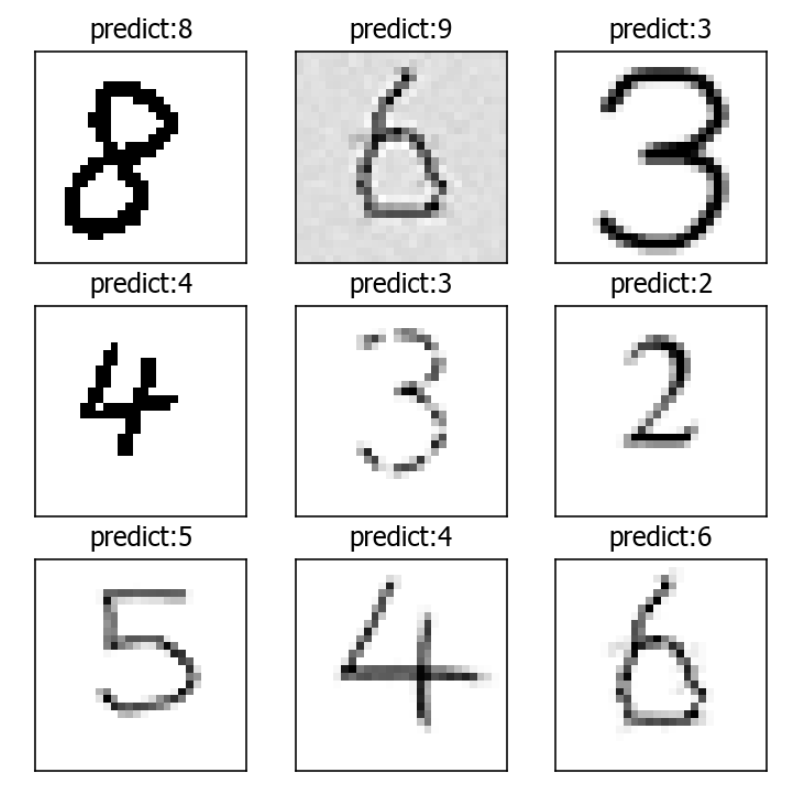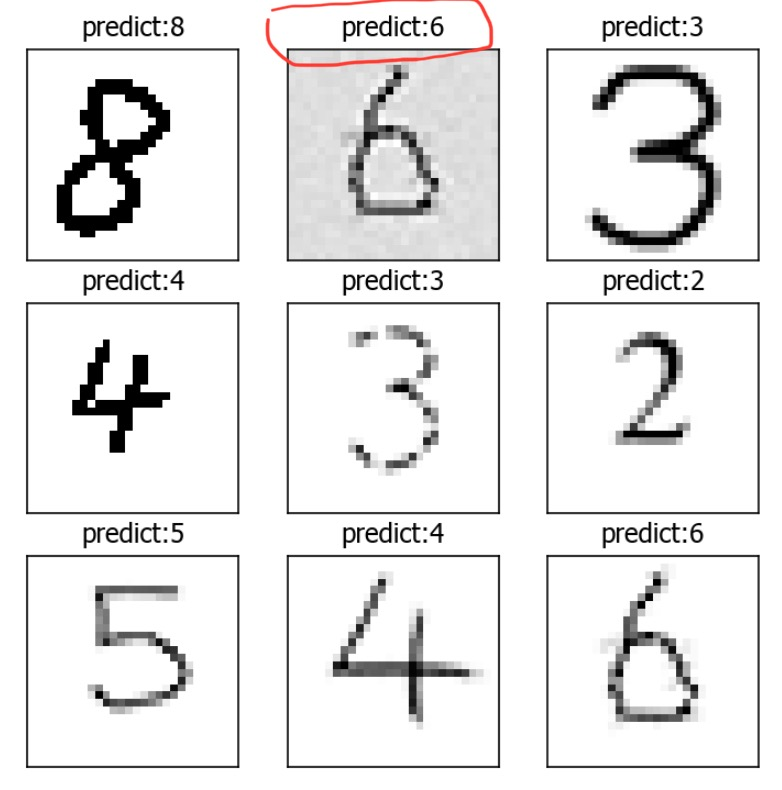# Anders Wang

### 1、什么是神经网络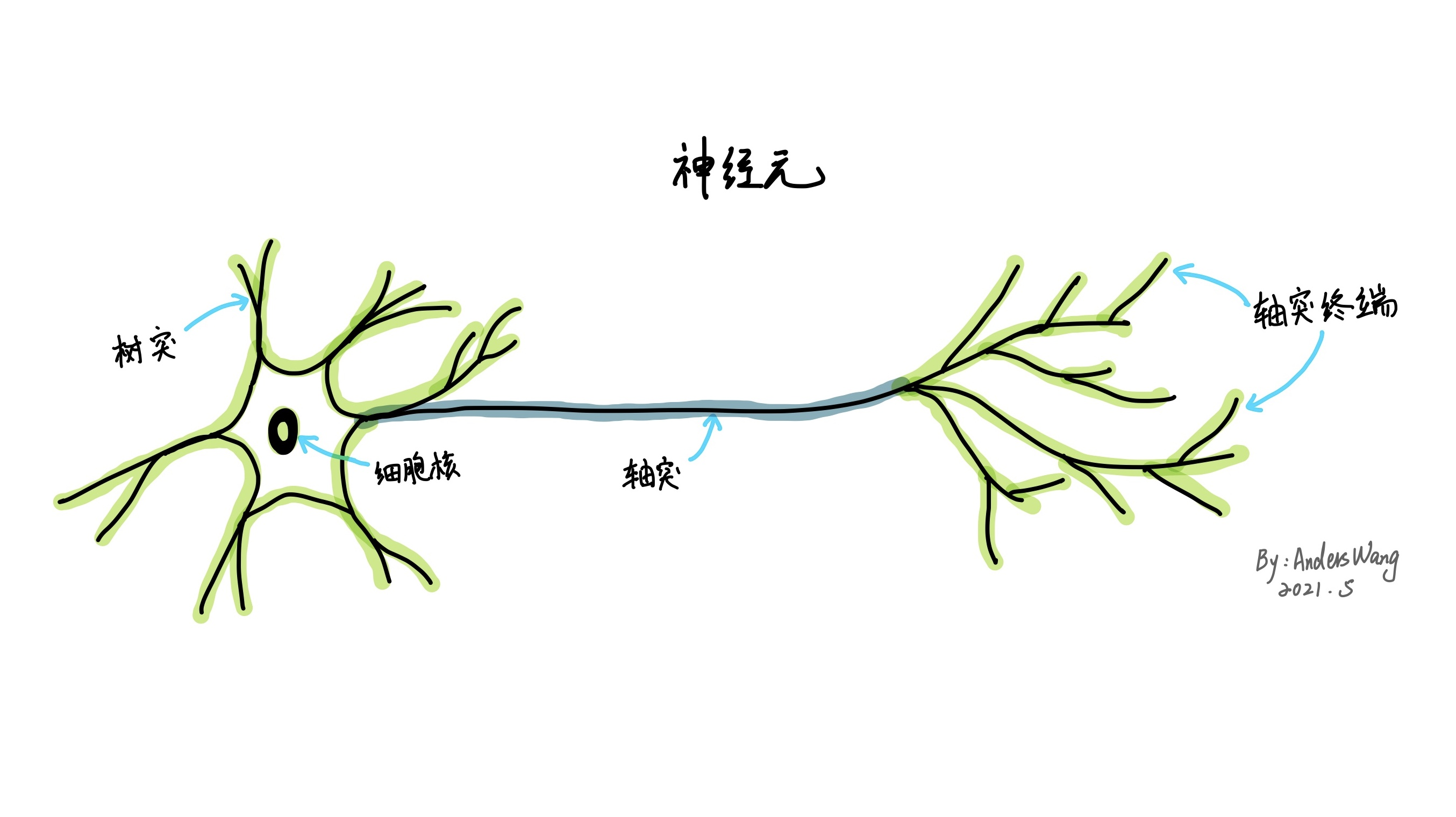### 2、人工神经网络大致流程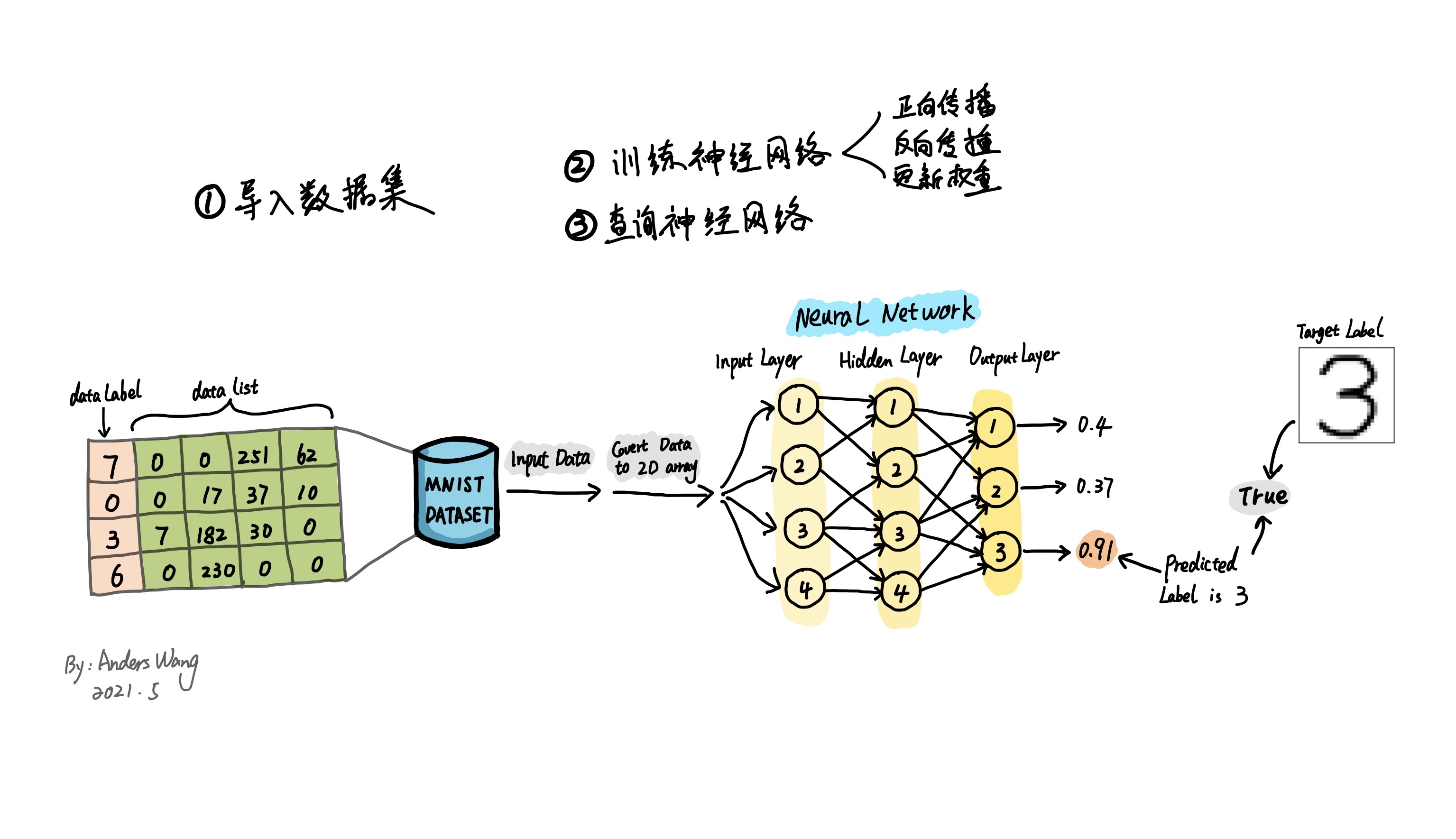### 3、准备MNIST数据集

MNIST 数据集是著名的手写图像集合，常用来测量和比较机器学习算法的性能。该数据集包括了用于训练机器学习模型的 60,000 个图像和用于测试性能的 10,000 个图像。现成的MNIST的数据集主要是CSV文件，它的格式如下图分解图所示。每一行由一连串数字分别以逗号隔开。其中第一个值是数据标签，代表图像的实际"数字"，比如第一个值为 2那这个图像应该被识别为 2。随后的一连串数值由逗号分隔代表手写数字的组成像素值，由于像素的尺寸是28*28=784，所以除去首位标签值后，还有一共784个值。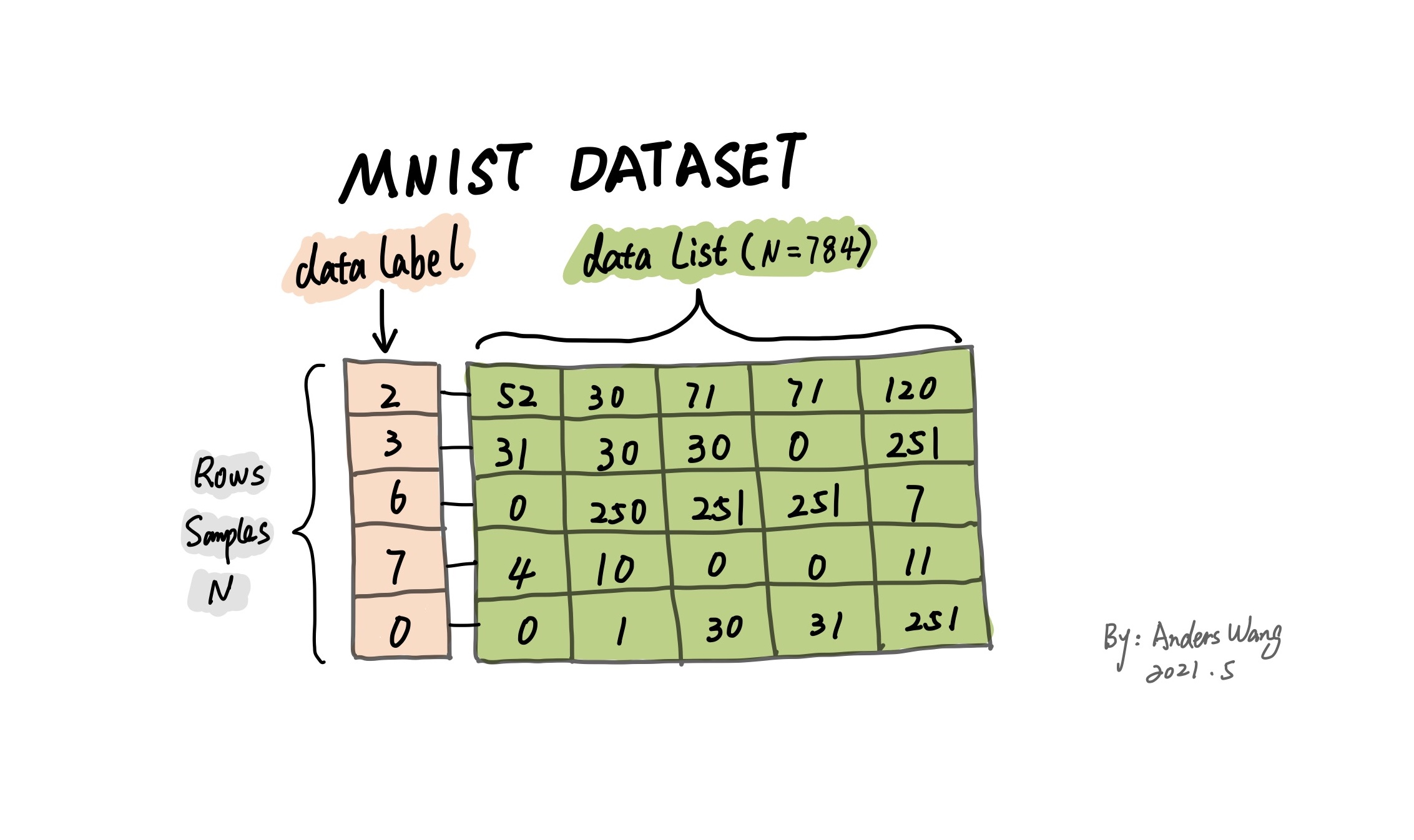### 4、神经网络的运行基本原理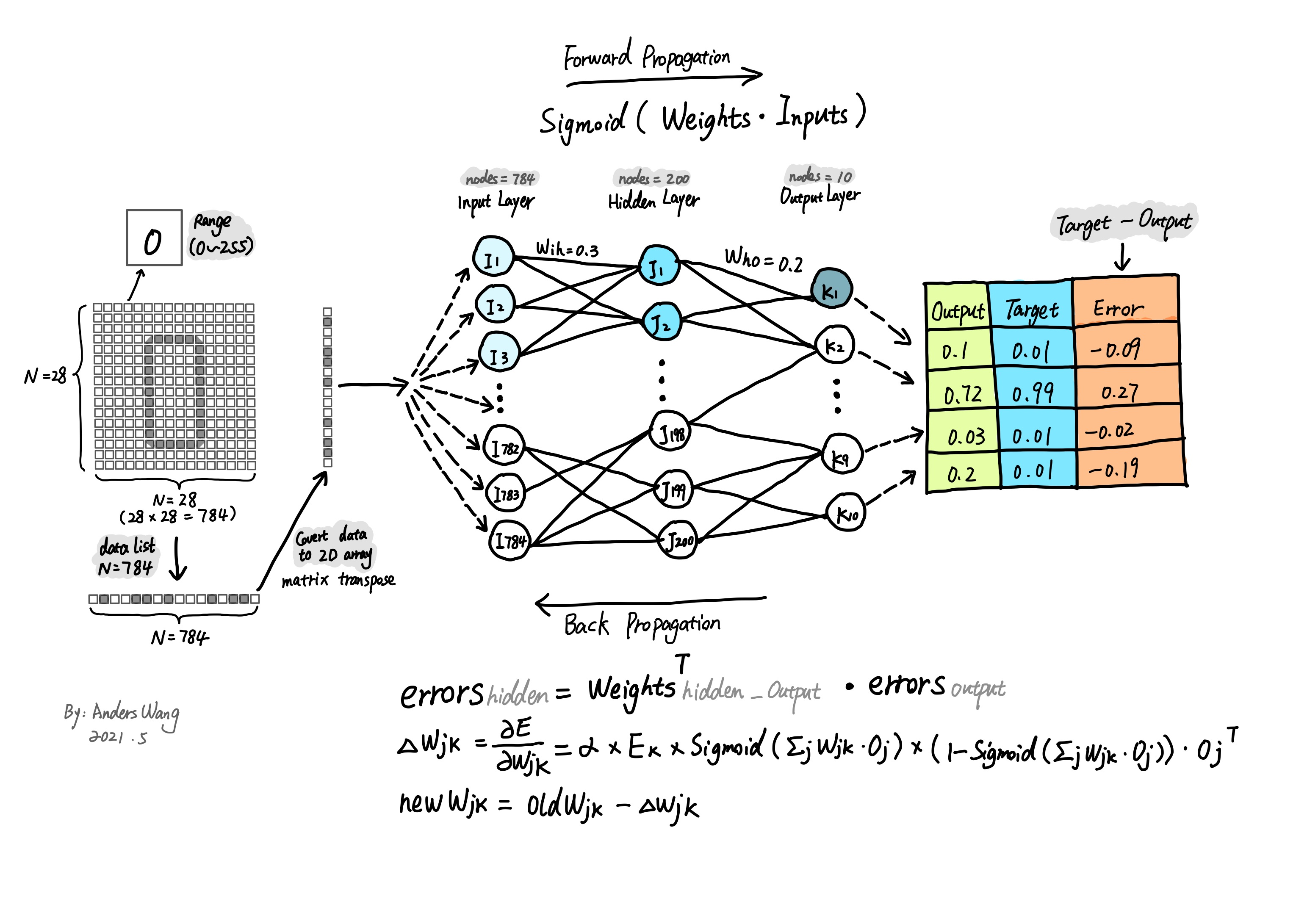### 4.1、神经网络的计算过程 --- 信号正向传播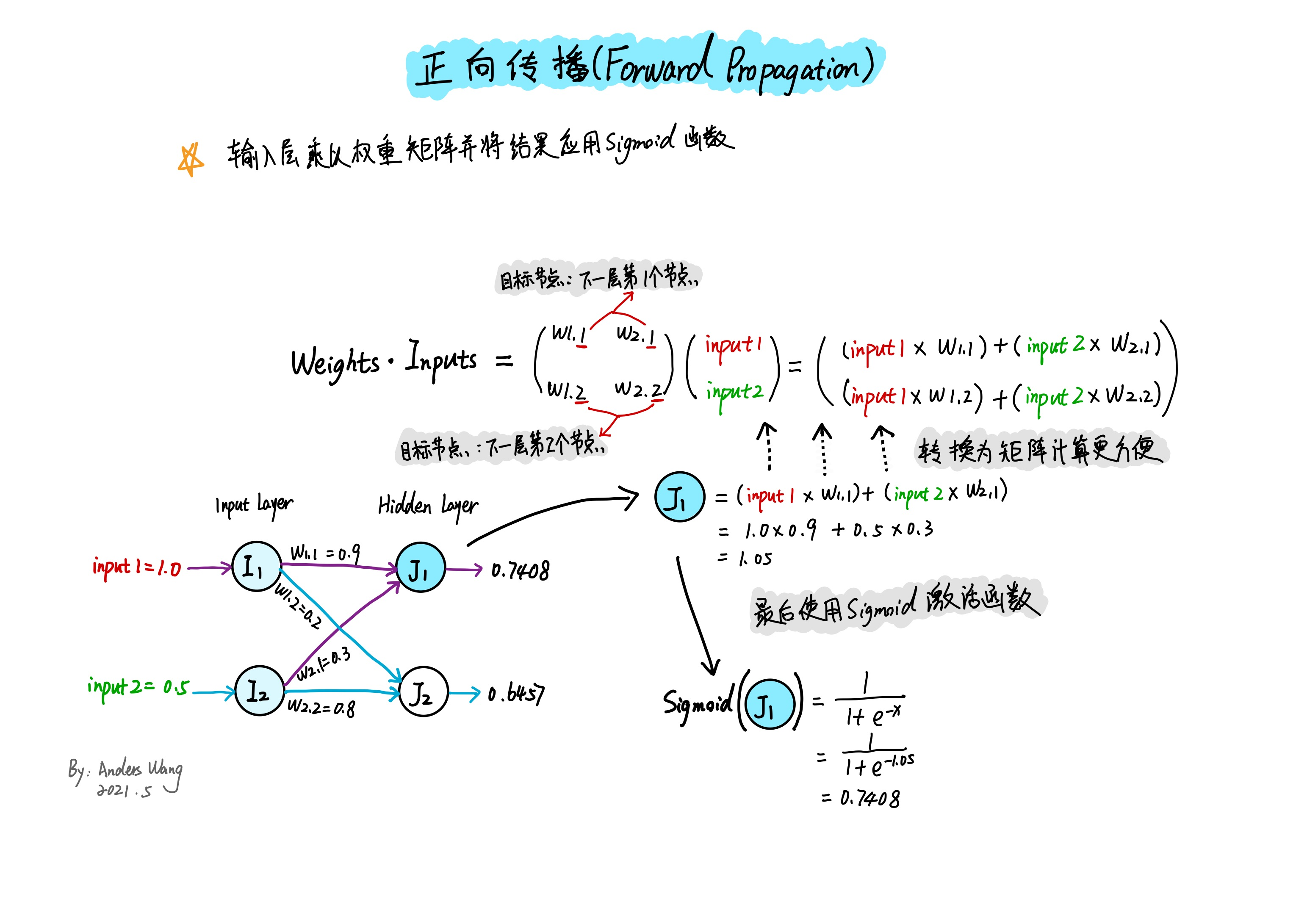### 4.2、神经网络的计算过程 --- 误差反向向传播

（1）直接将误差值以链接权重的个数N来平分，如输出层的误差值是由2个链接对应的节点组成，所以就以$\frac{1}{2}$分割误差值：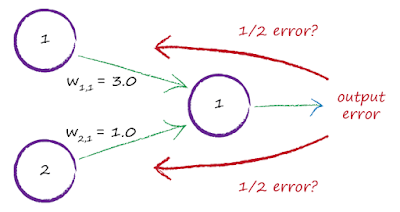（2）采用以链接权重值的占比来计算：

（3）直接用误差与链接权重相乘 $E_ {hidden}=W_ {i,j}^T⋅E_ {output}$（这也是本文使用的方法）。（4）与第3种方式相近，但是表达式额外除以了对应的链接权重个数N：

$E_ {hidden}=\frac{W_ {i,j}^T}{N}.E_ {output}$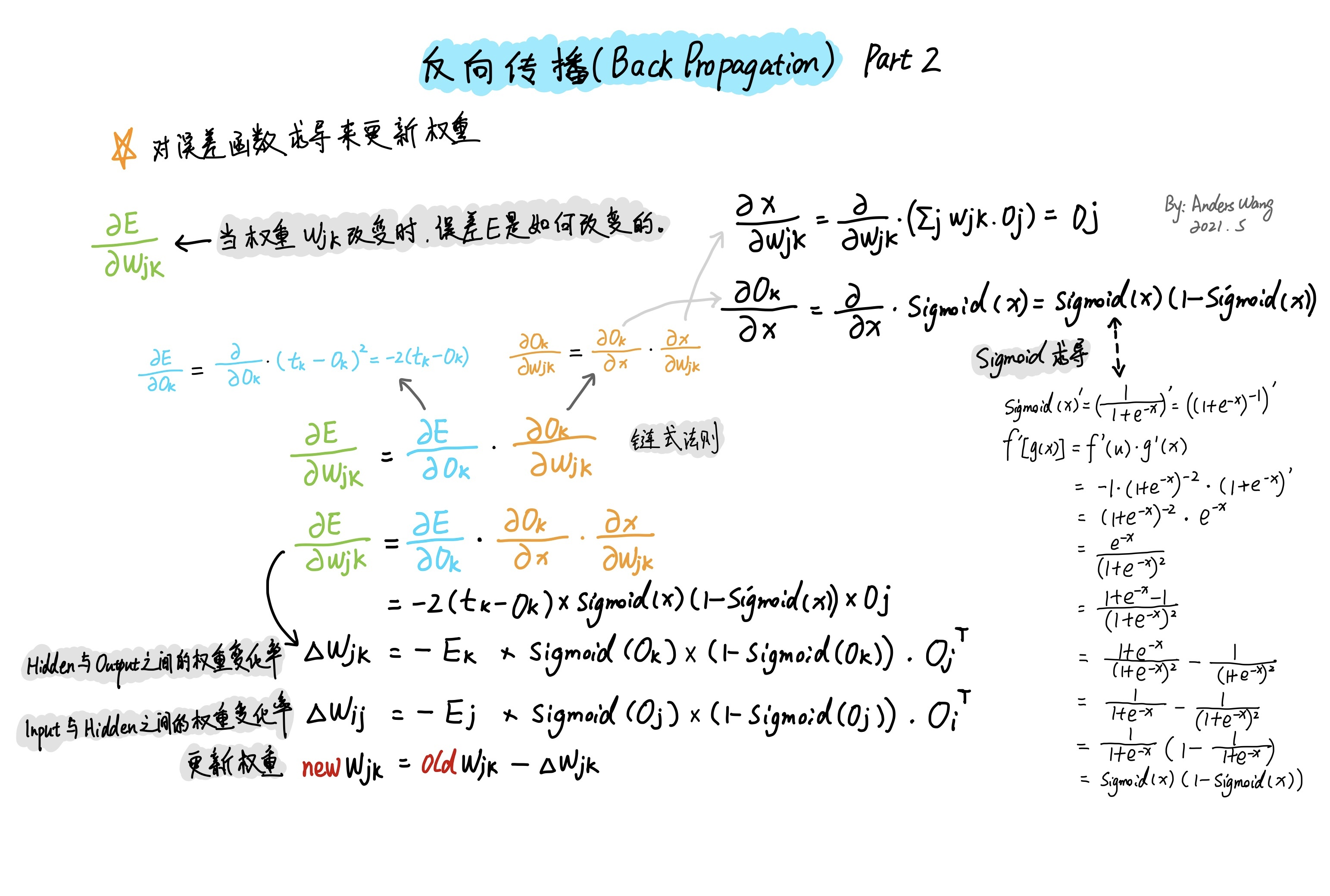$newW_ {jk}=oldW_ {jk}-\Delta{W_ {jk}}$

$newW_ {jk}=oldW_ {jk}+\alpha\times\Delta{W_ {jk}}$

#### Python代码实现

import numpy as np
import pandas as pd
import matplotlib.pyplot as plt
import scipy.special

from scipy import stats

np.random.seed(80)


_init_()方法：定义神经网络的基本参数，其中分别设置了输入层、隐藏层、输出层的节点数参数，以及输入层与隐藏层的权重值、隐藏层与输出层的权重值、学习率、激活函数。

train()方法：由于神经网络的训练模式组成是先通过正向传播得到基本误差，然后反向传播使用误差更新权重。所以在该方法里分别要实现正反向传播操作的过程。

query()方法：经过之前的一系列训练后，神经网络的连接权重值都已经优化到最佳数值，所以对于查询方法，只需要传入数据集进行一遍正向传播就可以输出结果。

### neural network class definition ###
class NeuralNetwork:
### initialise the neural network elements
def __init__(self, input_nodes, hidden_nodes, output_nodes, learning_rate):
self.i_nodes = input_nodes
self.h_nodes = hidden_nodes
self.o_nodes = output_nodes
self.lr = learning_rate

self.wih = np.random.normal(0.0, pow(self.h_nodes, -0.5), (self.h_nodes, self.i_nodes))
self.who = np.random.normal(0.0, pow(self.o_nodes, -0.5), (self.o_nodes, self.h_nodes))

# activation function is the sigmoid function
self.activation_function = lambda x: scipy.special.expit(x)

### training the neural network
def train(self, inputs_list, targets_list):
# convert the list to 2d array, because the each layer of data format must be 2D
inputs = np.array(inputs_list, ndmin = 2).T
targets = np.array(targets_list, ndmin = 2).T

# calculate the signals into hidden layer
hidden_inputs = np.dot(self.wih, inputs)
# calculate the signals emerging from hidden layer
hidden_outputs = self.activation_function(hidden_inputs)

# calculate the signals into output layer
final_inputs = np.dot(self.who, hidden_outputs)
# calculate the signals emerging from final layer
final_outputs = self.activation_function(final_inputs)

# error is the (target - actual):
output_errors = targets - final_outputs

# hidden layer error is the output_errors, split by weights, recombined at hidden nodes
hidden_errors = np.dot(self.who.T, output_errors)

# update the weights for the links between the hidden and output layers
self.who += self.lr * np.dot((output_errors * final_outputs * (1.0 - final_outputs)), np.transpose(hidden_outputs))
# update the weights for the links between the hidden and input layers
self.wih += self.lr * np.dot((hidden_errors * hidden_outputs * (1.0 - hidden_outputs)), np.transpose(inputs))

### query the neural network
def query(self, inputs_list):
# covert inputs list to 2d array
inputs = np.array(inputs_list, ndmin = 2).T

# calculate signals into hidden layer
hidden_inputs = np.dot(self.wih, inputs)
# calculate signals emerging from hidden layer
hidden_outputs = self.activation_function(hidden_inputs)

# calculate signals into output layer
final_inputs = np.dot(self.who, hidden_outputs)
# calculate signals emerging from output layer
final_outputs = self.activation_function(final_inputs)

return final_outputs


### training the neural network ###
training_data_file = open("neuralnetwork/mnist_dataset/mnist_train.csv", 'r')
training_data_file.close()

# define the number of input, hidden, output nodes and learning rate
input_nodes = 784
hidden_nodes = 200
output_nodes = 10
learning_rate = 0.1

# create the instance of neural network
n = NeuralNetwork(input_nodes, hidden_nodes, output_nodes, learning_rate)

# epochs is the number of times the training data set is used for training
epochs = 5

for e in range(epochs):
# go through all records in the traning data set
for record in training_data_list:
# split the record by the ',' commas
all_values = record.split(',')

# scale and shift the inputs
inputs = (np.array(all_values[1:], dtype = np.float) / 255.0 * 0.99) + 0.01

# create the target output values(because we use the sigmoid function,
# its range is 0<s<1, all 0.01, expect the desired label which is 0.99)
targets = np.zeros(output_nodes) + 0.01

# all_values is the target label for this record
targets[int(all_values)] = 0.99
# training the model
n.train(inputs, targets)

## create rotated variations in order to increase the sample capacity
# rotated anticlockwise by x degrees
inputs_plusx_img = scipy.ndimage.interpolation.rotate(inputs.reshape(28,28), 10, cval=0.01, order=1, reshape=False)
# training the model
n.train(inputs_plusx_img.reshape(784), targets)

# rotated clockwise by x degrees
inputs_minusx_img = scipy.ndimage.interpolation.rotate(inputs.reshape(28,28), -10, cval=0.01, order=1, reshape=False)
# training the model
n.train(inputs_minusx_img.reshape(784), targets)


### test the neural network ###
test_data_file = open("neuralnetwork/mnist_dataset/mnist_test.csv", "r")
test_data_file.close()

# scorecard for how well the network performs, initially empty
scorecard = []

# go through all the records in the test data set
for record in test_data_list:
# split the record by the ',' commas
all_values = record.split(',')

# convert answer is first label
correct_label = int(all_values)

inputs = (np.array(all_values[1:], dtype = np.float) / 255.0 * 0.99) + 0.01

## query the neural network
outputs = n.query(inputs)

# the index of the highest value corrsponds to the label
predict_label = np.argmax(outputs)

if (predict_label == correct_label):
scorecard.append(1)
else:
scorecard.append(0)

# calculate the performance score, the fraction of correct answers
scorecard_array = np.array(scorecard)
print(f'performance: {round(scorecard_array.sum() / scorecard_array.size * 100, 2)}%')


performance: 96.65%


### test the custom image ###
from pathlib2 import Path
import imageio
import matplotlib.pyplot as plt

img_array_list = []

files_path = Path.cwd().joinpath('neuralnetwork/my_own_images')
if files_path.exists():
for file in files_path.iterdir():
if file.match('*.png'):
img_array = imageio.imread(str(file), as_gray = True)
img_array_list.append(255.0 - img_array)

fig, axes = plt.subplots(figsize=(6, 6), nrows = 3, ncols = 3)
i = 0

for row in range(3):
for col in range(3):
# reshape the 784 based on white ground
img_data = img_array_list[i].reshape(784)
# scale and shift the inputs
inputs = (img_data / 255.0 * 0.99) + 0.01
# query the neural network
outputs = n.query(inputs)

# the index of the highest value corresponds to the target label
label = np.argmax(outputs)

axes[row][col].imshow(img_array_list[i], cmap = 'Greys', interpolation = 'None')
axes[row][col].set_title(f'predict:{label}')
axes[row][col].set_xticks([])
axes[row][col].set_yticks([])

i += 1